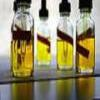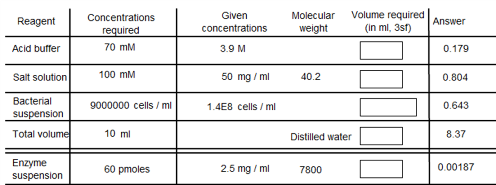You may also likeMathematical Issues for Chemists

A brief outline of the mathematical issues faced by chemistry students.Reaction Rates

Explore the possibilities for reaction rates versus concentrations with this non-linear differential equationCatalyse That!

Can you work out how to produce the right amount of chemical in a temperature-dependent reaction?

Concentrations

Age 16 to 18 Challenge Level:The trick to solving this question is to work slowly and break the problem down into smaller calculations.

The total volume is 10ml, and the required concentration of acid is 70mM. Therefore, the number of moles of acid needed are: $$0.01 \times 70 \times 10^{-3} = 7\times 10^{-4}$$

We have a given concentration of 3.9M, and so the volume necessary is: $$V = \frac{7\times 10^{-4}}{3.9} =0.179 ml.$$

For the salt solution, we want to obtain a concentration of 100 mM. Since the final volume is 10 ml, the number of moles required is: $$0.01 \times 100 \times 10^{-3} = 1 \times 10^{-3}$$

However, the given concentration is 50 mg /ml. This can be converted into a molar concentration by dividing by the molecular weight, which gives 1.24 mmol / ml, which is the same as 1.24 $\times$ 10$^{-3}$ mol / ml. The volume required can be calculated by: $$V = \frac{1 \times 10^{-3}}{1.24 \times 10^{-3}} = 0.804 ml$$

The bacterial suspension requires a concentration of 9000000 cells / ml, and since the final volume is 10ml, then the number of cells required is: $$9000000 \times 10 = 9 \times 10^7 cells$$

The given concentration of the suspension is 1.4 $\times$ 10$^8$ cells /ml, and so the amount of this suspension needed is : $$V = \frac{9 \times 10^7}{1.4 \times 10^8} = 0.643 ml$$

The enzyme suspension needs an amount of 60 pmoles /ml. Thus the total numer of moles needed is 600 pmoles. Also the given concentration is 2.5 mg/ml, which can be converted to a molar concentration by dividing by the molecular weight: $$\frac{2.5}{7800} = 3.21 \times 10^{-4} mmol/ml$$

The volume required is given by dividing the number of required moles by the concentration: $$\frac{600 \times 10^{-12}}{3.21 \times 10^{-7}} = 1.87 \times 10^{-3}$$

Summing together the four required volumes gives 1.63ml, and so to make this up to the required volume of 10ml requires the addition of 8.37 ml of water.